# Electronics and Communication Engineering - Microwave Communication

### Exercise :: Microwave Communication - Section 2

31.

Which of the following terminations makes the input impedance of a line equal to characteristic impedance Z0?

 A. line open circuited at far end B. line terminated in Z0 C. line short circuited at far end D. line terminated in inductance

Answer: Option B

Explanation:

If ZL is load impedance and Z0 is characteristic impedance.

Input impedance =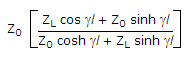. If ZL = Z0 the input impedance = Z0.

32.

In microwave system the function of mode filter is

 A. to suppress modes with lower cut off frequencies B. to suppress modes with higher cut off frequencies C. to change mode of wave transmission D. both (b) and (c)

Answer: Option D

Explanation:

Mode filter suppress modes with lower cutoff frequencies.

33.

For the strip line in the given figure the capacitance per unit length =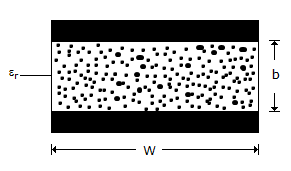A.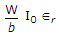B.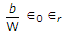C.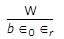D.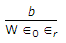Answer: Option A

Explanation: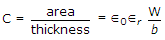frads/unit length.

34.

The total field developed by an antenna array at a distant point is

 A. phasor sum of fields produced by individual antennas of the array B. algebraic sum of fields produced by individual antennas of the array C. either (a) or (b) depending on type of array D. neither (a) nor (b)

Answer: Option A

Explanation:

Since field is a phasor quantity we have to take phasor sum.

35.

High speed logic circuits use

 A. pulses with very small width B. pulses with very large width C. pulses whose width is neither small nor large D. either (b) or (c)

Answer: Option A

Explanation:

High speed means time period is small. Therefore pulses should also have small width.

#### Current Affairs 2021

Interview Questions and Answers